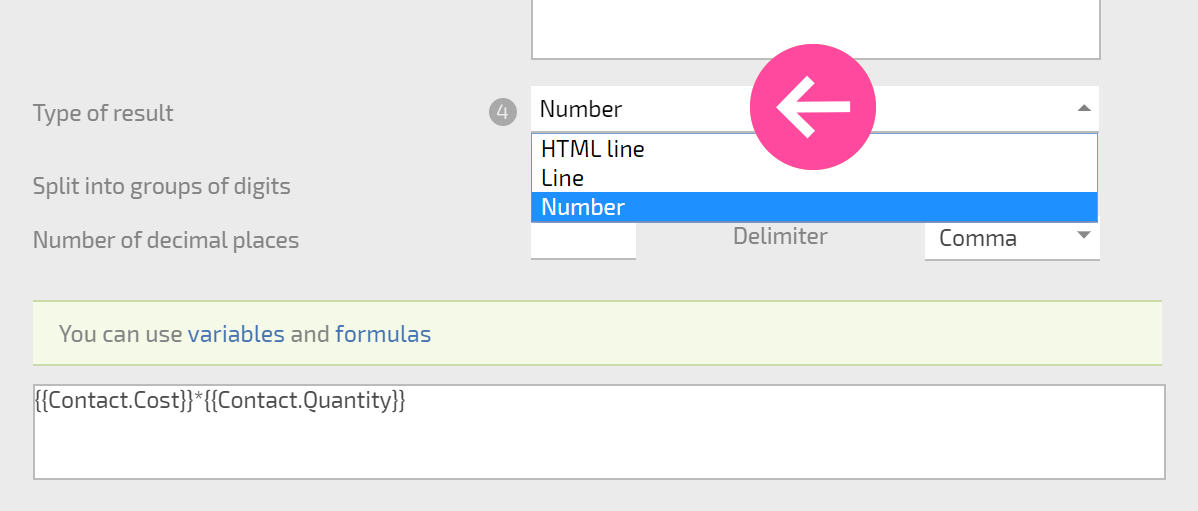# Calculated contact fields

A calculated field is a custom contact field that lets you calculate a value you need, using data from other Planfix contact fields and standard Planfix functions.

## Calculated field settings

The key component of a calculated field is the formula used to calculate its value:You can also choose the format in which the value of your calculated field will be stored:### Important

• Calculated fields are calculated when a contact is created or modified. If the formula uses a value from a field in a different object (such as system date) and the field in the other object changes, the value in the calculated field will not change. It will only be recalculated when a contact parameter is changed that calls the Contact modified event. You must keep this in mind when you use fields from other system objects.
• Your plan determines the maximum number of calculated fields you can add.